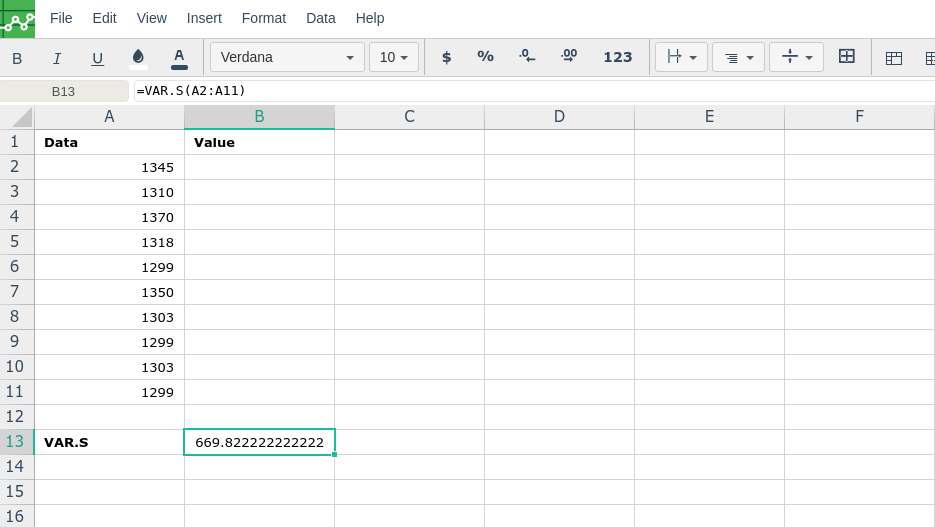# VAR.S

### Definition

Estimates variance based on a sample (ignores logical values and text in the sample).

### Syntax

VAR.S(number1,[number2],...)

The VAR.S function syntax has the following arguments:

• Number1     Required. The first number argument corresponding to a sample of a population.

• Number2, ...     Optional. Number arguments 2 to 254 corresponding to a sample of a population.

### Remarks

• VAR.S assumes that its arguments are a sample of the population. If your data represents the entire population, then compute the variance by using VAR.P.

• Arguments can either be numbers or names, arrays, or references that contain numbers.

• Logical values, and text representations of numbers that you type directly into the list of arguments are counted.

• If an argument is an array or reference, only numbers in that array or reference are counted. Empty cells, logical values, text, or error values in the array or reference are ignored.

• Arguments that are error values or text that cannot be translated into numbers cause errors.

• If you want to include logical values and text representations of numbers in a reference as part of the calculation, use the VARA function.

• VAR.S uses the following formula:

where x is the sample mean AVERAGE(number1,number2,…) and n is the sample size.

###Error.

User does not have sufficient privileges to access this Content